• Matlab 最优化工具箱;...若要计算一个函数 f (x) 的最大值可根据f (x) 的最大值= - f (x) 的最小值得到 目前所有的最优化算法均不能保证出全局最优解即整个定义域上的最值以下介绍的命令实际上是极小值局部最小
• 线性规划问题   在一组线性约束条件下的限制下，一线性目标函数最大或最小的问题。 线性规划标准型 数学标准型： 可行解：满足约束条件的解矩阵x=...Matlab线性规划函数——linprog >> help linprog linpr
线性规划问题
在一组线性约束条件下的限制下，求一线性目标函数最大或最小的问题。线性规划标准型

数学标准型：可行解：满足约束条件的解矩阵x=[x1,x2,x3,…,xn]。
最优解：是目标函数达到最大值或者最小值的可行解。
可行域：所有可行解构成的集合称为问题的可行解，记为R。

matlab标准型：f,x,b,beq,lb,ub为列向量
f称为价值向量
b称为资源向量
A，Aeq为矩阵

Matlab线性规划函数——linprog
>> help linprog
linprog - Solve linear programming problems

This MATLAB function solves min f'*x such that A*x ≤ b.

x = linprog(f,A,b)
x = linprog(f,A,b,Aeq,beq)
x = linprog(f,A,b,Aeq,beq,lb,ub)
x = linprog(f,A,b,Aeq,beq,lb,ub,options)
x = linprog(problem)
[x,fval] = linprog(___)
[x,fval,exitflag,output] = linprog(___)
[x,fval,exitflag,output,lambda] = linprog(___)

linprog 的参考页


x:返回决策向量的取值
fval返回目标函数的最优解
f为价值向量
A和b对应线性不等式约束
Aeq和beq对应线性等式约束
lb和ub分别对应决策向量的下界向量和上界向量
options是控制参数

例题讲解题目分析：
这里求解的最优解是最大值，而matlab里面的标准函数是求最小值为最优解，因此需要对题目进行变形。另外，对最优解的约束同样需要进行整理称为matlab的使用形式。整理结果如下：Matlab****程序实现
f=[-2;-3;5];
a=[-2,5,-1;1,3,1];
b=[-10;12];
aeq=[1,1,1];
beq=7;
[x,y]=linprog(f,a,b,aeq,beq,zeros(3,1));
x,y=-y				%由于之前将求max最优解变形称为了min，这里的y要求相反的值

输出结果：
x=3x1
6.4286
0.5714
0
y=14.5714题目分析
这个题目里面求的最优解为最小值，因此不需要进行转换，只需要对约束条件进行转换即可。在约束条件中，只有线性不等式约束以及决策向量下界向量，没有线性等式约束。转换结果如下：程序实现
f=[2;3;1];    %价值向量是列向量
a=[1,4,2;3,2,0];
b=[8;6];
aeq=[];
beq=[];
[x,y]=linprog(f,-a,-b,aeq,beq,zeros(3,1))	%一定要注意这里的符号


特别说明：一定要记住matlab的线性规划标准函数的形式，标准形式里面只有小于等于号，如果程序出现大于号一定要进行变换！！！


展开全文• min cTx （求最大值用-c） s.t.{Ax≤b（不等式约束条件）（当出现>时，把对应行系数乘以负号即可）Aeqx=beq（等式约束条件）lb≤x≤ub（x上下界） s.t. \begin{cases} Ax \leq b （不等式约束条件）（当出现>...
matlab规定了线性规划的标准形式为：

min cTx （求最大值用-c）
$s.t. \begin{cases} Ax \leq b （不等式约束条件）（当出现>时，把对应行系数乘以负号即可）\\ Aeqx = beq （等式约束条件）\\ lb \leq x \leq ub （x上下界） \end{cases}$

c，x，b，beq，lb，ub为列向量，A，Aeq为矩阵，c称为价值向量，b称为资源向量。
matlab里面的命令为：

[x,fval] = linprog(c,A,b)

[x,fval] = linprog(c,A,b,Aeq,beq)

[x,fval] = linprog(c,A,b,Aeq,beq,lb,ub)

x返回的是决策向量的取值，fval返回的是目标函数的最优解。


展开全文数学建模 算法
• 线性规划(Linear Programming LP)问题是在一组线性约束条件的限制下，一线性目标函数最大或最小的问题。...它的标准形式求得都是最小值，若是最大值要转化为最小值 matlab函数为 [x, fval] = linprog(f
线性规划(Linear Programming LP)问题是在一组线性约束条件的限制下，求一线性目标函数最大或最小的问题。
在matlab中，线性规划的标准形式如下
$\begin{cases} Ax\le b \\ aeq·x=beq \\ lb\le x\le ub \end{cases}$
它的标准形式求得都是最小值，若是最大值要转化为求最小值
matlab函数为
[x, fval] = linprog(f, A, b, aeq, beq, lb, ub, options)

这里的各参数对应上的公式，举个例子来说明
$max\quad z=2x_1+3x_2-5x_3$
$\begin{cases} x_1+x_2+x_3=7 \\ 2x_1-5x_2+x_3\ge 10 \\ x_1+3x_2+x_3\le 12 \\ x_1,x_2,x_3\ge 0 \end{cases}$
求解代码：
clc,clear;
f = [2; 3; -5];
a = [-2 5 -1; 1 3 1];
b = [-10; 12];
aeq = [1 1 1];
beq = 7;
lb = zeros(3, 1);
%//f取-f意为取反的最小值
[x, fval] = linprog(-f, a, b, aeq, beq, lb);
fprintf('x1=%.4f, x2=%.4f, x3=%.4f\nz=%.4f\n', x, -fval);

结果为：
x1=6.4286, x2=0.5714, x3=0.0000
z=14.5714



展开全文数学建模
• matlab用多元非线性规划logistic函数因变量的最大值，自变量有3个，都是维数为809的向量，其中两个自变量的具体值已给出。 目标函数： function p=fun1(x) [A]=xlsread('********.xls'); w=A(:,1); ...c++ c语言
• 定义 如果目标函数或约束条件中至少有一个是非线性函数时的最优化问题就叫做非线性规划问题 其它情况: 目标函数的最大值或约束条件为小于等于零的情况都可通过取其相反数化为上述一般形式1二次规划用MATLAB软件...
• 一、线性规划1.1 定义它的原理很简单，跟高中学过的简单线性规划一样，不多说1.2 MATLAB标准形式线性规划的目标函数可以是求最大值，也可以是求最小值，约束条件的不等号可以 是小于号也可以是大于号。为了避免这种...
一、线性规划1.1 定义它的原理很简单，跟高中学过的简单线性规划一样，不多说1.2 MATLAB标准形式线性规划的目标函数可以是求最大值，也可以是求最小值，约束条件的不等号可以 是小于号也可以是大于号。为了避免这种形式多样性带来的不便，Matlab 中规定线性规划的标准形式为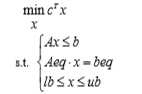其中c和 x为n 维列向量， A、 Aeq 为适当维数的矩阵，b 、beq为适当维数的列向量。二、线性规划模型1、运输问题某商品有m 个产地、n个销地，各产地的产量分别为a1,a2..am，各销地的需求量分别b1,b2..bn。若该商品由i产地运到 j 销地的单位运价为cij ，问应该如何调 运才能使总运费最省？(1)产销平衡即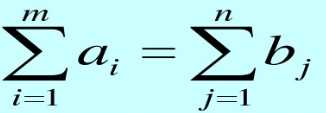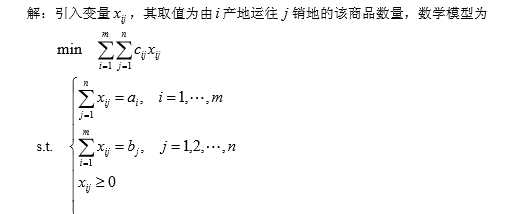(2)产销不平衡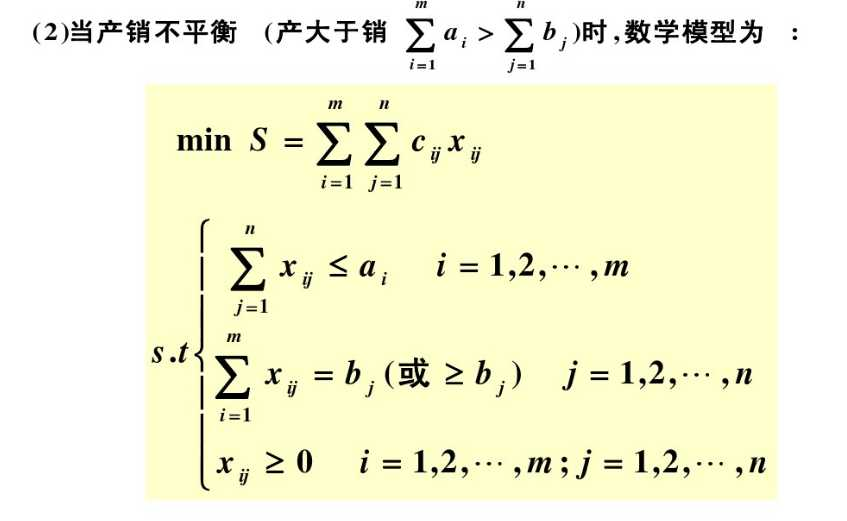2、指派问题拟分配n人去干n项工作，每人干且仅干一项工作，若分配第i人去干第 j 项工作，需花费 cij 单位时间，问应如何分配工作才能使工人花费的总时间最少？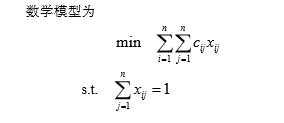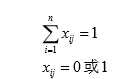上述指派问题的可行解可以用一个矩阵表示，其每行每列均有且只有一个元素为 1，其余元素均为 0***指派问题的匈牙利算法，见书，很简单三、对偶理论与灵敏度分析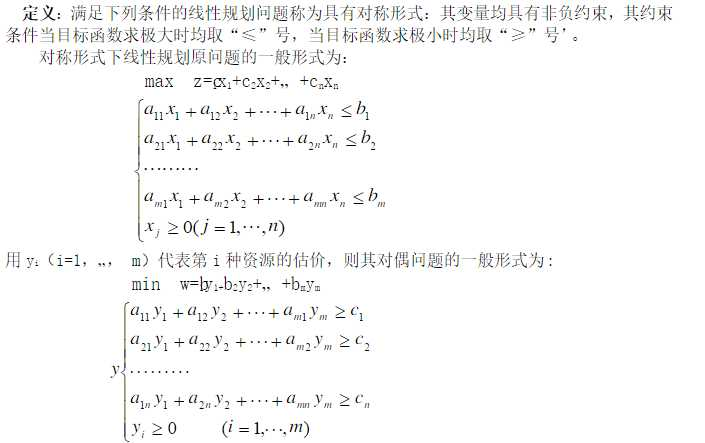原文：http://www.cnblogs.com/nefu929831238/p/6287530.html
展开全文算法 矩阵
• 一元无约束规划 [x, fval]= fminbnd (f,x1,x2）其中fun为目标函数，支持字符...例1 函数在区间【0，8】上的最大值、最小值。 代码 如下 y='2*exp(-x)*sin(x)' [xmin,fmin]=fminbnd(y,0,8) %fplot(y,[0,8]) 答...
• 目标函数可以使求最大值，也可以是求最小值，约束条件的不等号可以使小于号也可以是大于号。 为了避免以上形式多样性带来的不便，Matlab中线性规划的标准模为： 基本函数形式为linprog(c,A,b)，它的返回值是.....
• 今天学习了一些matlab线性规划相关的知识 A*B’: '和T都是转置的意思。 A.B: 必须保证A、B形状一样，同为mn矩阵。 C=A(i,j)B(i,j) AB： 与线性代数里的矩阵相乘计算方法一样，不需A、B形状一样，只需满足A的列数与B...
• matlab遗传算法求最大值问题例题完整代码_IT认证_资格考试/认证_教育专区。matlab遗传算法求最大值问题例题完整代码 本文件的目的是减少您打字的烦恼 %主程序:用......11MATLAB求函数极值_数学_自然科学_专业资料。...
• LINGO的语法规定 1目标函数的最大值或最小值分别用MAX=或MIN=来表示 2每个语句必须以分号结束每行可以有许多语句语句可以跨行 3变量名称必须以字母(A~Z)开头由字母数字(0~9)和下划线所组成长度不超过32个字符不...
• 线性规划的目标函数可以是求最大值，也可以是求最小值，约束条件的不等号可以是小于号也可以是大于号。可以使用同一个函数 ，只需变号就行了。 求最小值的标准式如下： 其中，min c^Tx是目标函数，s.t.大括号就是...python 数学建模
• 线性规划的目标函数可以是求最大值，也可以是求最小值，约束条件的不等号可以是小于号也可以是大于号。为了避免这种形式多样性带来的不方便，matlab规定线性规划的标准形式为： 它有两种常用形式： X = ...
• 线性规划问题 线性规划是数学规划中的一类最简单规划问题，常见的线性规划是一个有约束的，...由于MATLAB中求解的是目标函数是最小值的问题，但如果我们的目标函数是求最大值，可以通过对目标函数中每一项中乘以...
• 第一章线性规划 §1 线性规划 ...·求最大值线性规划问题转换： 基本函数形式为 linprog(c,A,b)，它的返回值是向量 x 的值。还有其它的一些函数调用形 式（在 Matlab 指令窗运行 help linprog 可以看到所有的
• 2.线性规划matlab标准形式线性规划的目标函数可以求最大值也可以求最小值，约束条件的不等号可以是小于号也可以是大于号，因此在matlab中给出了统一形式。其中c和x均为n维列向量，A、Aeq为适当维数（列数与x维数...
• 在一组线性的等式或不等式下的约束下，一线性目标函数最大值或最小值的问题。 二、Matlab 中规定线性规划的标准形式 其中 c 和 x 为 n 维列向量， A 、 Aeq 为适当维数的矩阵，b 、beq 为适当维数的列向量。 基本...
• 线性规划的目标函数可以是求最大值，也可以是求最小值，约束条件的不等号可以 是小于号也可以是大于号。 为了避免这种形式多样性带来的不便，Matlab 中规定线性规划的标准形式为 其中c和 x为n 维列向量， A、 ...
• 在一组⎡线性⎦约束条件限制下，一⎡线性⎦目标函数的最大值和最小值。 线性规划问题的主要难点在于模型的建立，若想快速准确地建立模型，合理选取决策变量是关键。 &2.线性规划matlab形式 Matlab规定的线性...数学建模
• 线性规划问题是在一组线性约束条件的限制下，一线性目标函数最大或最小的问题。 2、Matlab 中规定线性规划的标准形式 min cx Ax<=b; Aeq.x=beq; lb<=x<=ub 3、基本函数形式 linprog(c,A,b)，它的...算法
• 线性整数规划就是对于非线性函数f最优解时自变量的，其中要求自变量必须是整数 例题： 某厂向用户提供发动机，合同规定，第一、二、三季度末分别交货40台、60台、80台．每季度的生产费用为 （元），其中x是该...
• ​ 理论上，蒙特卡洛法可以解决所有规划问题，但由于其只能求解近似解，而对于线性规划、特殊的非线性规划和特殊的整数规划，可以用对应的函数出精确解，所以蒙特卡洛法适用于无法出精确解的大部分非线性规划和...矩阵 算法
• 注意matlab中求的是“最大值”、“小于等于”， 如果我们求最大值或者约束条件中为”大于等于“， 只需要在前加负号 上述有错，f为价值向量 注意对照线性规划模型的标准式：“最小、“小于等于” zeros(3,1)：...
• 我理解的线性规划就是在给定的一些线性方程可以列出的约束条件下求解目标函数的极值。...默认求最小值，求最大值的话，把c换成-c就好了（最大值取个﹣就是最小值了） 转载于:https://www.cnblogs.com/...
• 简介 线性规划（Linear programming,简称LP）是运筹学中研究较早、发展较快、应用广泛、方法较成熟的一个重要分支，它是辅助人们进行科学管理的一种数学方法。研究线性约束条件下线性目标函数的极值...若需求最大值数学建模
• 规划问题的求解原理 假如规划问题有有限个最优解，则一定有某个最优解是可行区域的一个极点。... 线性规划的目标函数可以是求最大值，也可以是求最小值，约束条件的不等号可以 是小于号也可以是大于号。为了避函数
• 将所有的约束条件和决策变量结合起来，解决求最大或最小值问题 用Matlab标准形式解决问题注意点 标准式中的 f，x，b，beq，lb，ub，均为列向量 只能求最小值，要求最大值则令f= - f，求出最小值，再将结果取...
• ## 使用 Matlab 解决数学建模问题

千次阅读 多人点赞 2018-12-06 00:35:37
线性规划函数1. 线性规划函数：linprog()1.1....线性规划的目标函数可以是求最大值，也可以是求最小值，约束条件的不等号可以是小于号也可以是大于号。 Matlab 中规定线性规划的标准形式为 min⁡xcTxs.t.{Ax≤...# matlab线性规划求最大值matlab 订阅